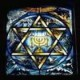## Answers: How Many Subnets/Hosts 2

The answers for the most recent subnetting speed practice, for “find the number of subnets and hosts question 2” are below the fold – FYI. Ask questions as needed.

The figure shows the big concept to find the number of network, subnet, and host bits. From there, it’s just doing calculations per the powers of 2.Here are the answers to this set of questions.

 Class Network Bits (N) Subnet Bits (S = P – N) Host Bits (32 – P) 1 A 8 14 10 2 A 8 1 23 3 B 16 8 8 4 C 24 5 3 5 C 24 4 4
 Number of Subnets Number of Hosts 1 2^14 = 16,384 2^10 – 2 = 1022 2 2^1 = 2 2^23 – 2 = 8,388,606 3 2^8 = 256 2^8 – 2 = 254 4 2^5 = 32 2^3 – 2 = 6 5 2^4 = 16 2^4 – 2 = 14
Subscribe
Notify ofInline FeedbacksHi Wendell…at first glance Im asking myself what does the letter P stand for?

I agree the previous version of ICND1 subnetting chapter needed a rewrite.

Ive been tutoring in the Cisco Academy where I live, and I find the less info you throw at someone the better…at least at first. I just use this cheat sheet:

128 192 224 240 248 252 254 255
___ ___ ___ ___ ___ ___ ___ ___
128 64 32 16 8 4 2 1

2^ for each binary 1 going from left to right to get the networks,
2^-2 on the bottom line going right to left to get hosts (or just look
directly below the last “on” bit, e.g. 32 is below the 224.

Kevin…its never easy teaching it, I know now from having tried!. I learned by you book and, though it is a bit wordy, looking back after I “got it” it all made good sense and laid a solid foundation.

Peace BroTheophilus,
Love the name, by the way. Makes me yearn to re-read Luke…

P simply stands for the prefix style mask’s value, to make the comparisons and small math problems briefer. The figure is from the new book, by the way.

And thanks for the note. The publisher pays by the word, so it can get wordy. 😉 In all seriousness, part of the challenge is that no matter where you draw the line with a brief version of a subnetting chapter, it’s leaving out a subset of readers who needed more explanation for the light bulb to go off. Hopefully the segments of concept/process/examples/practice will draw out all those “ah ha” moments.
Wendellthank yo so much … you solved my biggest problem thanks again 🙂

3
0